# c++ vector like array1043

Hi I'm working on a mql5 program and need arrays like std::vector of c++

for example in my c++ code i have something like this:

```class Neuron;
typedef std::vector<Neuron> Layer;
class net{
private:
std::vector<Layer> m_layers; //m_layers[layer_num][neuronum]
}```

how can i implement this in mql5 ?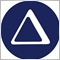Moderator
8188

pumper:

Hi I'm working on a mql5 program and need arrays like std::vector of c++

for example in my c++ code i have something like this:

how can i implement this in mql5 ?

Please take a look at this Article and also at the Documentation.1043

thanks for reply it helped but not completly.

the problem remains is that in vector as i represented in my example every dimension can have its own data type even class objects but here for a 2d array all dim's are same data type.?

any idea?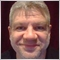41564

pumper:

thanks for reply it helped but not completly.

the problem remains is that in vector as i represented in my example every dimension can have its own data type even class objects but here for a 2d array all dim's are same data type.?

any idea?

Your question is unclear. You can use a dynamic array in mql5, but only the first dimension can be dynamic.

How is it related to "data type" ?1043

angevoyageur:

Your question is unclear. You can use a dynamic array in mql5, but only the first dimension can be dynamic.

How is it related to "data type" ?

If you look at my code example of c++ and explain me how to do something like this in mql5 its my answer.(many thanks)41564

pumper:
If you look at my code example of c++ and explain me how to do something like this in mql5 its my answer.(many thanks)
I already answer you : with a dynamic array.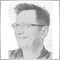1424

```class Neuron
{
public:
double n1;
};

struct Layer{
Neuron neurons[];
};

class net{
private:
Layer m_layers[]; //m_layers[layer_num][neuronum]
public:
net()
{
ArrayResize(m_layers,5);
ArrayResize(m_layers.neurons,5);
}

double Get(int l, int n)
{
return(m_layers[l].neurons[n].n1);
}

void Set(int l, int n, double val)
{
m_layers[l].neurons[n].n1=val;
}
};

net * mynet;
//+------------------------------------------------------------------+
//| Script program start function                                    |
//+------------------------------------------------------------------+
void OnStart()
{
mynet = new net();
mynet.Set(0,3,3.14);
Print(mynet.Get(0,3));
}
```
Something similar to this... I think . Regards Uwe1043

ugo58:
Something similar to this... I think . Regards Uwe

thanks thats what exactly I looking for

and one more question : what performance comparition is in using c++ dll's instead of using fully mql5 EA's?1424

Regarding perfomance that is difficult to judge.

You could use GetTickCount to measure the running time of  a complex part of code.

As with most systems the input/output of data is limiting factor for the design.

Interesting read for multi tasking:

https://www.mql5.com/en/articles/197

Regards  Uwe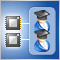Parallel Calculations in MetaTrader 5
• 2011.03.02
• Andrew
• www.mql5.com
Time has been a great value throughout the history of mankind, and we strive not to waste it unnecessarily. This article will tell you how to accelerate the work of your Expert Advisor if your computer has a multi-core processor. Moreover, the implementation of the proposed method does not require the knowledge of any other languages besides MQL5.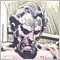5284

I landed on this thread late in the game, so I am sure this has been resolved.

The OP did say "like std::vector of c++," so the answers were correct.

But for posterity, this article describes when a linked list (e.g. CList) might be a better choice than a dynamic array (CDynamicArray).

https://stackoverflow.com/questions/393556/when-to-use-a-linked-list-over-an-array-array-list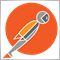2751

Anthony Garot:

I landed on this thread late in the game, so I am sure this has been resolved.

The OP did say "like std::vector of c++," so the answers were correct.

But for posterity, this article describes when a linked list (e.g. CList) might be a better choice than a dynamic array (CDynamicArray).

https://stackoverflow.com/questions/393556/when-to-use-a-linked-list-over-an-array-array-list

This is a good article and is why I have found CArrayObj to be much more efficient than CList in most cases as . This is how I use it.

`objvector<MyClass*> my_class_vector;`
Files:
objvector.mqh  1 kb